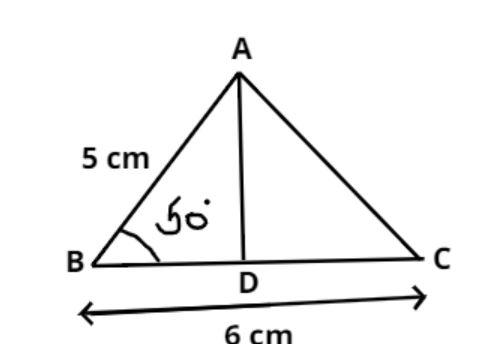Courses
Courses for Kids
Free study material
Free LIVE classes
MoreLIVE
Join Vedantu’s FREE Mastercalss

# Length of two sides of a triangle are $6cm$and$5cm$. If their included angle is$50^\circ$. What is the area of the triangle? Also find the length of its third side.$\left( {\sin 50^\circ = 0.77,\cos 50^\circ = 0.64,\tan 50^\circ = 1.19} \right)$Verified
365.1k+ views
Hint:Area of the triangle is half multiplied by its perpendicular times base.Pictorial representation of given problem is shown above
Let, AD is perpendicular to BC.
So, the area of the triangle is half multiply by its perpendicular times base
$= \dfrac{1}{2}\left( {AD} \right)\left( {BC} \right) = \dfrac{1}{2}\left( {AD} \right)\left( 6 \right) = 3AD$
Now in triangle ABD
$\sin 50^\circ = \dfrac{{AD}}{{AB}} = \dfrac{{AD}}{5} \\ \Rightarrow AD = 5\sin 50^\circ = 5 \times 0.77 = 3.85cm \\$
Therefore area of triangle is
Area$= 3AD = 3 \times 3.85 = 11.55c{m^2}$
Now in triangle ABD
$\tan 50^\circ = \dfrac{{AD}}{{BD}} = \dfrac{{3.85}}{{BD}} \\ \Rightarrow BD = \dfrac{{3.85}}{{\tan 50^\circ }} = \dfrac{{3.85}}{{1.19}} = 3.235cm \\ \Rightarrow DC = BC - BD = 6 - 3.235 = 2.765cm \\$
$\Rightarrow {\left( {AC} \right)^2} = {\left( {AD} \right)^2} + {\left( {DC} \right)^2} \\ \Rightarrow {\left( {AC} \right)^2} = {\left( {3.85} \right)^2} + {\left( {2.765} \right)^2} = 22.4677 \\ \Rightarrow AC = \sqrt {22.4677} cm \\$
So, the area of the triangle is $11.55c{m^2}$and the length of third side is$\sqrt {22.4677} cm$